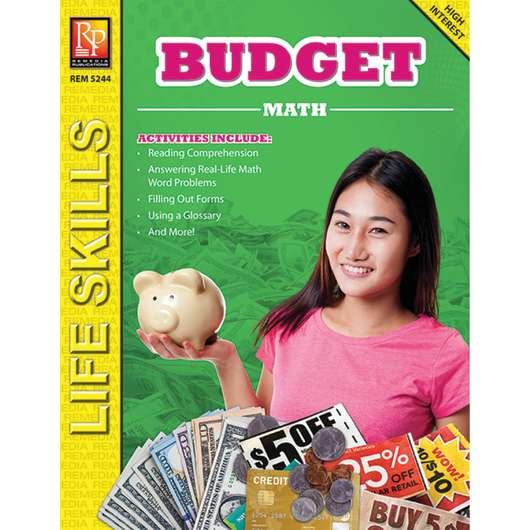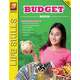•# Budget Math

Product Number: REM5244
\$10.99
A really practical application of math. Each book includes activities such as reading comprehension, filling out forms, using...
Quantity

A really practical application of math. Each book includes activities such as reading comprehension, filling out forms, using a glossary, and answering math word problems. The multi-step word problems cover adding and subtracting money, multiplying and dividing money, figuring percentages, working with large numbers, and more. This life skills program will help students master math skills that are essential to everyday life!

Introduce students to the vital role that money handling plays in all areas of a person’s life, while reinforcing basic math skills through a variety of problem-solving exercises. Comprehension, logical thinking, and sequential decisions are required to find answers to word problems relating to situations regarding making and using a budget. 64 pages.

REM5244